# Complier design

11 de Nov de 2021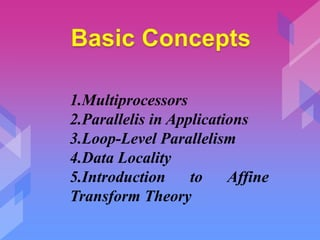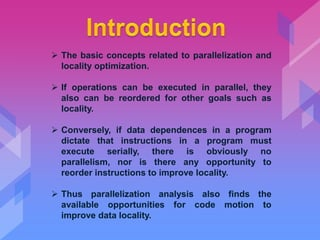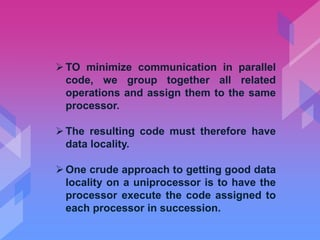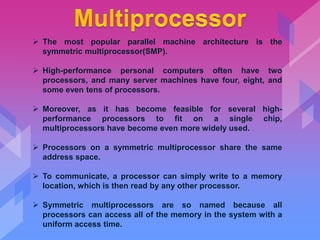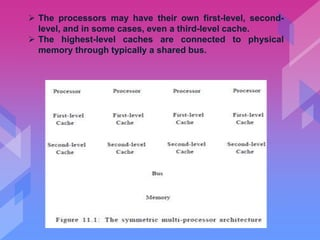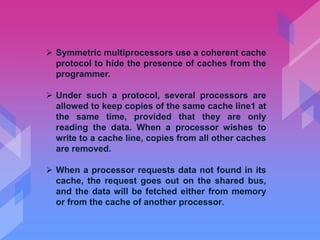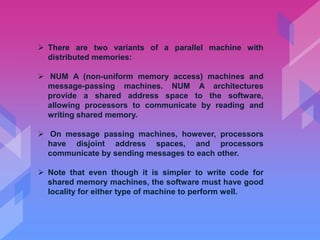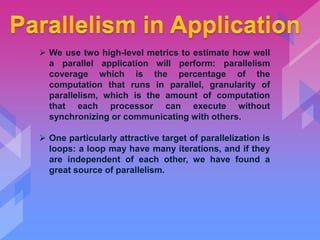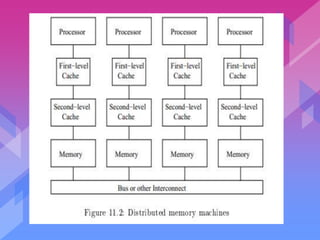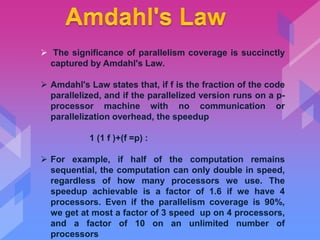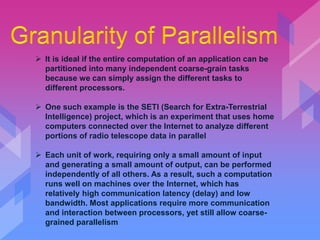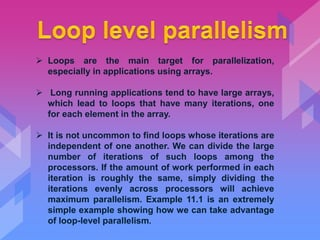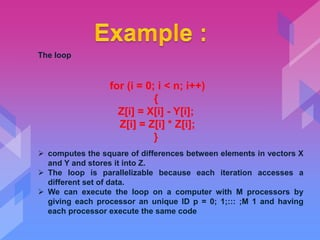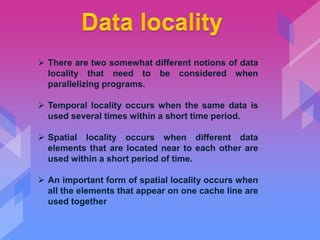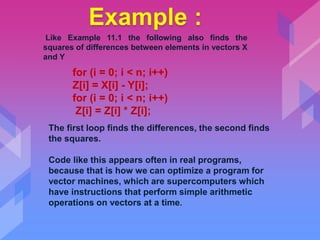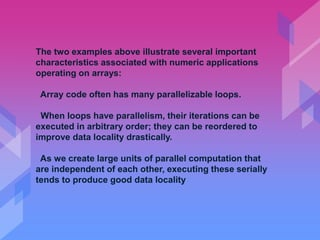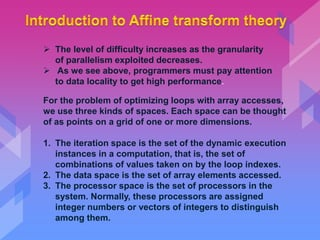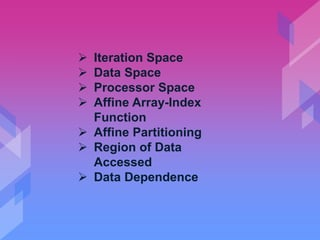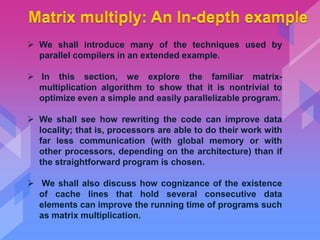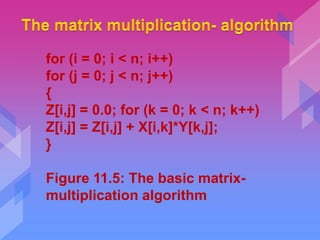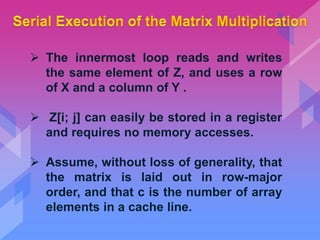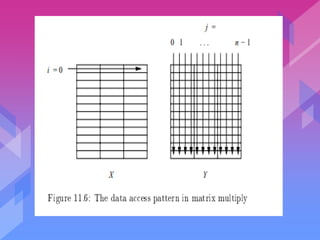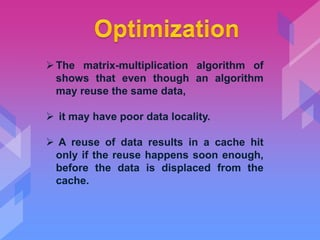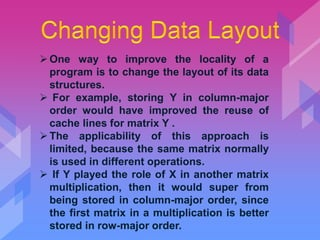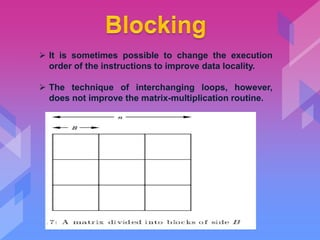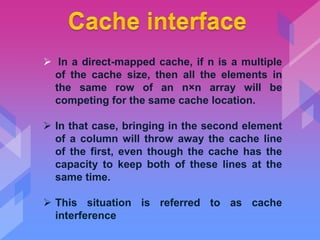1 de 30

### Complier design

• 2. ng for Parallelism and Locality
• 3. 1.Multiprocessors 2.Parallelis in Applications 3.Loop-Level Parallelism 4.Data Locality 5.Introduction to Affine Transform Theory
• 4.  The basic concepts related to parallelization and locality optimization.  If operations can be executed in parallel, they also can be reordered for other goals such as locality.  Conversely, if data dependences in a program dictate that instructions in a program must execute serially, there is obviously no parallelism, nor is there any opportunity to reorder instructions to improve locality.  Thus parallelization analysis also finds the available opportunities for code motion to improve data locality.
• 5. TO minimize communication in parallel code, we group together all related operations and assign them to the same processor. The resulting code must therefore have data locality. One crude approach to getting good data locality on a uniprocessor is to have the processor execute the code assigned to each processor in succession.
• 6.  The most popular parallel machine architecture is the symmetric multiprocessor(SMP).  High-performance personal computers often have two processors, and many server machines have four, eight, and some even tens of processors.  Moreover, as it has become feasible for several high- performance processors to fit on a single chip, multiprocessors have become even more widely used.  Processors on a symmetric multiprocessor share the same address space.  To communicate, a processor can simply write to a memory location, which is then read by any other processor.  Symmetric multiprocessors are so named because all processors can access all of the memory in the system with a uniform access time.
• 7.  The processors may have their own first-level, second- level, and in some cases, even a third-level cache.  The highest-level caches are connected to physical memory through typically a shared bus.
• 8.  Symmetric multiprocessors use a coherent cache protocol to hide the presence of caches from the programmer.  Under such a protocol, several processors are allowed to keep copies of the same cache line1 at the same time, provided that they are only reading the data. When a processor wishes to write to a cache line, copies from all other caches are removed.  When a processor requests data not found in its cache, the request goes out on the shared bus, and the data will be fetched either from memory or from the cache of another processor.
• 9.  There are two variants of a parallel machine with distributed memories:  NUM A (non-uniform memory access) machines and message-passing machines. NUM A architectures provide a shared address space to the software, allowing processors to communicate by reading and writing shared memory.  On message passing machines, however, processors have disjoint address spaces, and processors communicate by sending messages to each other.  Note that even though it is simpler to write code for shared memory machines, the software must have good locality for either type of machine to perform well.
• 10.  We use two high-level metrics to estimate how well a parallel application will perform: parallelism coverage which is the percentage of the computation that runs in parallel, granularity of parallelism, which is the amount of computation that each processor can execute without synchronizing or communicating with others.  One particularly attractive target of parallelization is loops: a loop may have many iterations, and if they are independent of each other, we have found a great source of parallelism.
• 12.  The significance of parallelism coverage is succinctly captured by Amdahl's Law.  Amdahl's Law states that, if f is the fraction of the code parallelized, and if the parallelized version runs on a p- processor machine with no communication or parallelization overhead, the speedup 1 (1 f )+(f =p) :  For example, if half of the computation remains sequential, the computation can only double in speed, regardless of how many processors we use. The speedup achievable is a factor of 1.6 if we have 4 processors. Even if the parallelism coverage is 90%, we get at most a factor of 3 speed up on 4 processors, and a factor of 10 on an unlimited number of processors
• 13.  It is ideal if the entire computation of an application can be partitioned into many independent coarse-grain tasks because we can simply assign the different tasks to different processors.  One such example is the SETI (Search for Extra-Terrestrial Intelligence) project, which is an experiment that uses home computers connected over the Internet to analyze different portions of radio telescope data in parallel  Each unit of work, requiring only a small amount of input and generating a small amount of output, can be performed independently of all others. As a result, such a computation runs well on machines over the Internet, which has relatively high communication latency (delay) and low bandwidth. Most applications require more communication and interaction between processors, yet still allow coarse- grained parallelism
• 14.  Loops are the main target for parallelization, especially in applications using arrays.  Long running applications tend to have large arrays, which lead to loops that have many iterations, one for each element in the array.  It is not uncommon to find loops whose iterations are independent of one another. We can divide the large number of iterations of such loops among the processors. If the amount of work performed in each iteration is roughly the same, simply dividing the iterations evenly across processors will achieve maximum parallelism. Example 11.1 is an extremely simple example showing how we can take advantage of loop-level parallelism.
• 15. The loop for (i = 0; i < n; i++) { Z[i] = X[i] - Y[i]; Z[i] = Z[i] * Z[i]; }  computes the square of differences between elements in vectors X and Y and stores it into Z.  The loop is parallelizable because each iteration accesses a different set of data.  We can execute the loop on a computer with M processors by giving each processor an unique ID p = 0; 1;::: ;M 1 and having each processor execute the same code
• 16.  There are two somewhat different notions of data locality that need to be considered when parallelizing programs.  Temporal locality occurs when the same data is used several times within a short time period.  Spatial locality occurs when different data elements that are located near to each other are used within a short period of time.  An important form of spatial locality occurs when all the elements that appear on one cache line are used together
• 17. for (i = 0; i < n; i++) Z[i] = X[i] - Y[i]; for (i = 0; i < n; i++) Z[i] = Z[i] * Z[i]; Like Example 11.1 the following also finds the squares of differences between elements in vectors X and Y The first loop finds the differences, the second finds the squares. Code like this appears often in real programs, because that is how we can optimize a program for vector machines, which are supercomputers which have instructions that perform simple arithmetic operations on vectors at a time.
• 18. The two examples above illustrate several important characteristics associated with numeric applications operating on arrays: Array code often has many parallelizable loops. When loops have parallelism, their iterations can be executed in arbitrary order; they can be reordered to improve data locality drastically. As we create large units of parallel computation that are independent of each other, executing these serially tends to produce good data locality
• 19.  The level of difficulty increases as the granularity of parallelism exploited decreases.  As we see above, programmers must pay attention to data locality to get high performance. For the problem of optimizing loops with array accesses, we use three kinds of spaces. Each space can be thought of as points on a grid of one or more dimensions. 1. The iteration space is the set of the dynamic execution instances in a computation, that is, the set of combinations of values taken on by the loop indexes. 2. The data space is the set of array elements accessed. 3. The processor space is the set of processors in the system. Normally, these processors are assigned integer numbers or vectors of integers to distinguish among them.
• 20.  Iteration Space  Data Space  Processor Space  Affine Array-Index Function  Affine Partitioning  Region of Data Accessed  Data Dependence
• 22.  We shall introduce many of the techniques used by parallel compilers in an extended example.  In this section, we explore the familiar matrix- multiplication algorithm to show that it is nontrivial to optimize even a simple and easily parallelizable program.  We shall see how rewriting the code can improve data locality; that is, processors are able to do their work with far less communication (with global memory or with other processors, depending on the architecture) than if the straightforward program is chosen.  We shall also discuss how cognizance of the existence of cache lines that hold several consecutive data elements can improve the running time of programs such as matrix multiplication.
• 23. for (i = 0; i < n; i++) for (j = 0; j < n; j++) { Z[i,j] = 0.0; for (k = 0; k < n; k++) Z[i,j] = Z[i,j] + X[i,k]*Y[k,j]; } Figure 11.5: The basic matrix- multiplication algorithm
• 24.  The innermost loop reads and writes the same element of Z, and uses a row of X and a column of Y .  Z[i; j] can easily be stored in a register and requires no memory accesses.  Assume, without loss of generality, that the matrix is laid out in row-major order, and that c is the number of array elements in a cache line.
• 26. The matrix-multiplication algorithm of shows that even though an algorithm may reuse the same data,  it may have poor data locality.  A reuse of data results in a cache hit only if the reuse happens soon enough, before the data is displaced from the cache.
• 27. One way to improve the locality of a program is to change the layout of its data structures.  For example, storing Y in column-major order would have improved the reuse of cache lines for matrix Y . The applicability of this approach is limited, because the same matrix normally is used in different operations.  If Y played the role of X in another matrix multiplication, then it would super from being stored in column-major order, since the first matrix in a multiplication is better stored in row-major order.
• 28.  It is sometimes possible to change the execution order of the instructions to improve data locality.  The technique of interchanging loops, however, does not improve the matrix-multiplication routine.
• 29.  In a direct-mapped cache, if n is a multiple of the cache size, then all the elements in the same row of an n×n array will be competing for the same cache location.  In that case, bringing in the second element of a column will throw away the cache line of the first, even though the cache has the capacity to keep both of these lines at the same time.  This situation is referred to as cache interference